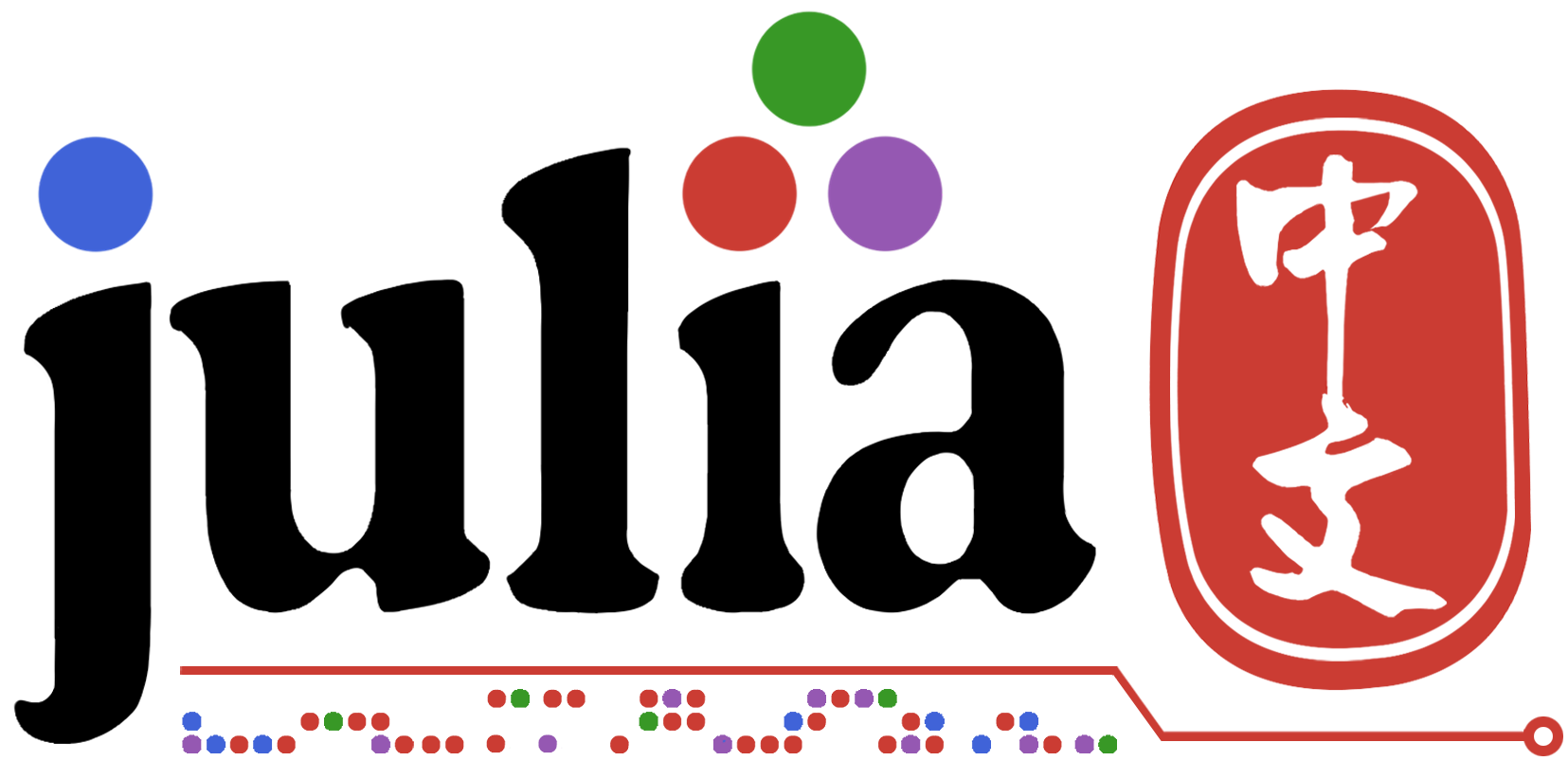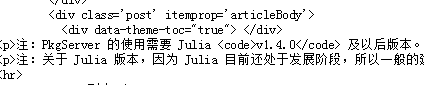# 一份简单的Plots官网文档总结

http://docs.juliaplots.org/latest/

### 1、Plots初识

#### 1.2 基本安装

##### 1.2.1 安装Plots

`import Pkg;Pkg.add("Plots")`

##### 1.2.2 自行安装后端包

`Pkg.add("GR")`

`Pkg.add("PyPlot")`

`Pkg.add("Plotly")`

##### 1.2.3 第一个画图

``````using Plots
x=1:10;y=rand(10,2)
p=plot(x,y)
z=rand(10)
plot!(p,x,z)
``````

• 默认设置：default(show=true)

• 声明后端时设置：gr(show=true)

• 函数display()或者display§

• 函数gui()或者gui§

• 函数plot(x,y,show=“true”)

##### 1.2.4 将结果保存成图片

• savefig(p,“img.png”)

• png(“img”)

• plot(rand(10),fmt=:png)

### 2、相关属性设置

#### 2.1 常用属性

##### 2.1.1 plot函数中设置

title 标题
label 图例 `label=["line1" "line 2"`]
xlabel X轴
ylabel Y轴
xticks X轴刻度 `xticks="0:1:10"`
yticks Y轴刻度
xlims X轴范围 `xlims=(1,20)`
ylims Y轴范围
linewidth 线条粗细 `lw=3`
linecolor 线条颜色 `lc=["blue" "green"]`
linestyle 线条风格 `ls=[:dash :dot]`
markercolor 点标志颜色 `mc=[:orange :purple]`
markershape 点标志形状 `shape=[:circle :star5]`
markersize 点标志大小 `ms=5`
fillcolor 填充颜色 `fc="red"`
background 背景颜色 `bg="white"`
##### 2.1.2 专门的属性函数设置

title!() title
xlabel!() xlabel
ylabel!() ylabel
xticks!/yticks!() xticks/yticks
xlims!/ylims!() xlims/ylims

#### 2.2 多功能属性设置

axis/xaxis/yaxis xlabel+xlims+xticks
line linestyle+linewidth+linecolor
fill fillrange+fillcolor
marker markershape+markersize+markercolor

#### 2.3 查看自己想要的属性

Plot包下的属性主要可分为四类：Series、Plots、SubPlot、Axis。可分别通过`plotattr(:Series)``plotattr(:Plot)``plotattr(:Subplot)``plotattr(:Axis)`查看该类主要提供哪些属性。再可以通过`plotattr("attrname")`了解具体属性的使用方法。

• Series类主要设置系列图相关的属性，比如线条颜色。
• Plot类主要设置画图相关的属性，比如画图窗口大小位置。
• Subplot类主要设置子图相关的属性，比如每个子图的图例。
• Axis类主要设置坐标轴相关的属性。

### 3、高级用法

#### 3.1 各种系列图

• plot()中通过seriestype属性指定。比如`seriestype=:scatter`，具体有哪些类型可通过`plotattr("seriestype")`查看。
• 可以直接调用类型函数。比如scatter()。

#### 3.2 子图与布局

``````plot(rand(10,4),layout=(2,2))
``````

``````p1=plot(rand(10))
p2=scatter(rand(10))
p3=histogram(rand(10))
p4=bar(rand(10))
plot(p1,p2,p3,p4,layout=4)
``````

grid函数举例：

``````plot(rand(100,4),layout=grid(4,1),heights=[0,1,0,4,0,4,0,1])
``````

``````l = @layout [
a{0.3w} [grid(3,3)
b{0.2h}  ]
]
plot(rand(10, 11),
layout = l,  seriestype = [:bar :scatter :path]
)
``````

#### 3.3 拓展基本使用（recipe）

##### 3.3.1 对数据类型拓展

``````using StatsPlots
using DataFrames
df = DataFrame(a = 1:10, b = 10 * rand(10), c = 10 * rand(10))
@df df plot(:a, [:b :c])
``````
##### 3.3.2 对特定类型拓展

``````using Distributions
plot(Normal(3, 5), lw = 3)
``````
##### 3.3.3 画图拓展

``````using RDatasets, StatsPlots
iris = dataset("datasets", "iris")
@df iris marginalhist(:PetalLength, :PetalWidth)
``````
##### 3.3.4 系列图拓展

``````y = rand(100, 4)
violin(["Series 1" "Series 2" "Series 3" "Series 4"], y, leg = false)
``````

#### 3.4 数据源

##### 3.4.1 矩阵

``````using Plots
xs=range(0, 2π, length = 10)
data=[sin.(xs) cos.(xs) 2sin.(xs) 2cos.(xs)]
labels=["Apples" "Oranges" "Hats" "Shoes"]
markershapes=[:circle :star5 :rect :+]
colors=[:green :orange :red :yellow]
plot(xs,data,label=labels,shape=markershapes,color=colors)
``````
##### 3.4.2 函数

``````using Plots
tmin = 0
tmax = 4π
tvec = range(tmin, tmax, length = 100)
plot(sin.(tvec), cos.(tvec))
``````

`plot(sin, cos, tvec)`
`plot(sin,cos,tmin,tmax)`

##### 3.4.3 DataFrame类型

``````using StatsPlots, RDatasets
iris = dataset("datasets", "iris")
@df iris scatter(
:SepalLength,
:SepalWidth,
group = :Species,
m = (0.5, [:+ :h :star7], 12),
bg = RGB(0.2, 0.2, 0.2)
)
``````

#### 3.5 颜色设置的高级用法

• 符号或者字符串。:red等价于"red"

• RGB颜色，这里默认RGB(A,B,C)里面用的是0-1之间的数，A=a/255、B=b/255、C=c/255。

• 一个整数，自动会选择相对应的颜色。

``````using Plots
p1=plot(rand(10),lc=:red)
p2=plot(rand(10),st=:scatter,lc=10)
p3=plot(rand(10),lc=RGB(0.1,0.2,0.3))
plot(p1,p2,p3,layout=(3,1))
``````

<各种颜色名称> 另外可以通过color_palette设置颜色，这时候color=:auto。palette的值可以借助Plots.ColorScheme，主要包括ColorVector、 ColorGradient。

8赞

github仓库：PyPlot 只调用的matplotlib.pyplot module？

1赞

bar 里边是不是不能画斜线啊